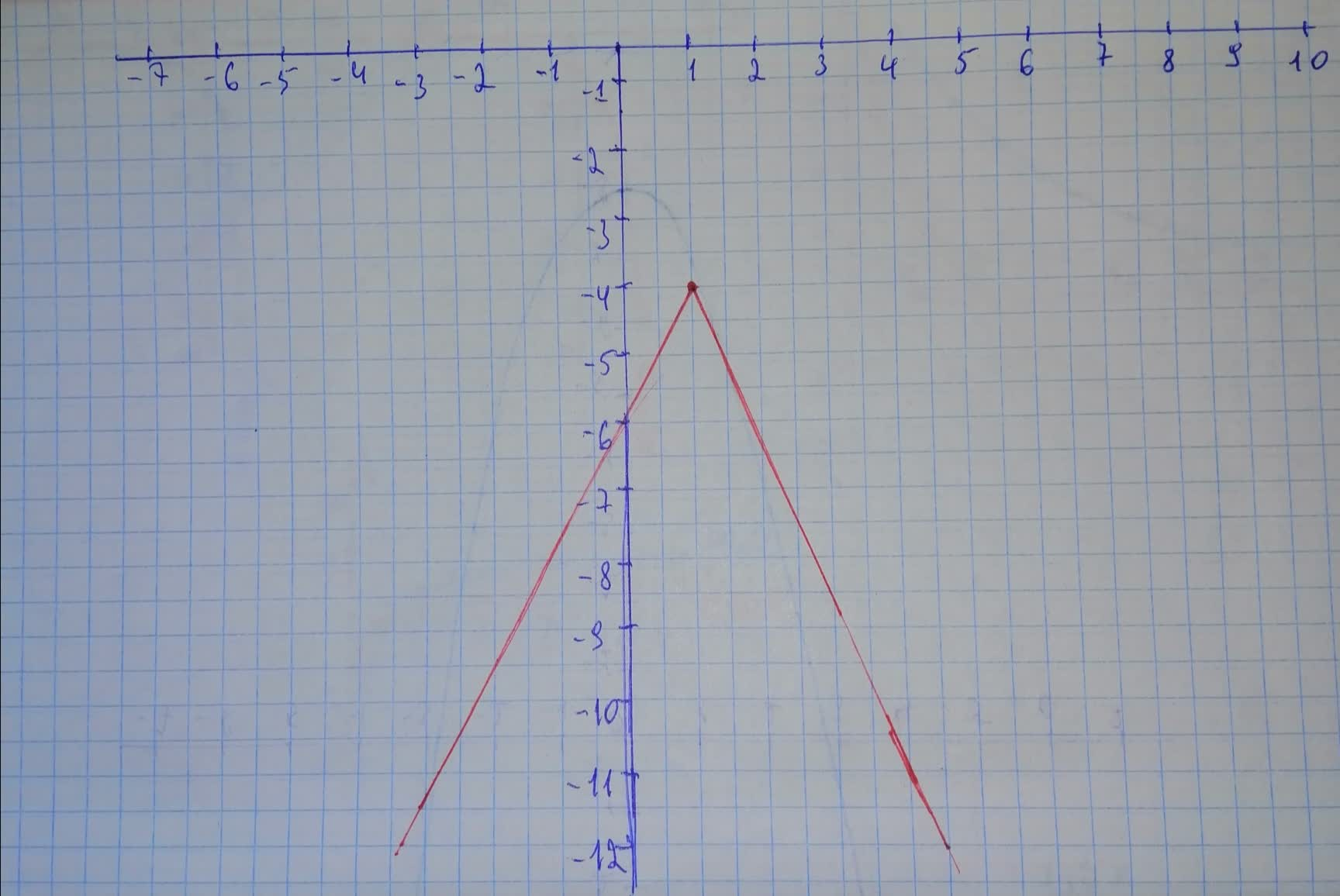# g is related to one of the six parent functions. a) Identify the parent function f. b) Describe the sequence of transformations from f to g. c) Sketch the graph of g by hand. d) Use function notation to write g in terms of the parent function f. g(x)= -2|x - 1| - 4Wotzdorfg 2021-01-10 Answered
g is related to one of the six parent functions.
a) Identify the parent function f.
b) Describe the sequence of transformations from f to g.
c) Sketch the graph of g by hand.
d) Use function notation to write g in terms of the parent function f.
You can still ask an expert for help

• Questions are typically answered in as fast as 30 minutes

Solve your problem for the price of one coffee

• Math expert for every subject
• Pay only if we can solve itYusuf Keller
a) Parent function: $f\left(x\right)=|x|$
b) Reflection in the y-axis
Vertical stretch by a factor of 2
Horizontal shift 1 unit to the right
Vertical shift 4 units downward
c) The graph will bed) In function notation,